# How to Convert Positive Numbers to Negative

This tutorial will teach you how to change positive numbers to negative with Paste special multiply operation in excel. How to convert positive number to negative or how to reverse the number signs with VBA code in Excel.

## Convert Positive Numbers to Negative with Paste Special Operation

To change positive numbers to negative in excel, you can do it with copy and paste, you need to copy a cell that contains a negative number -1, then select the cells that contain the positive numbers, and use the multiply operation on the Pasted Special menu. Then it will perform the multiplication on the selected cell. So all positive number will be change to negative.

You can do it as following steps:

1# type one negative number –1 in one cell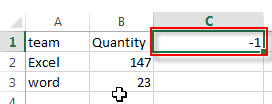2# select that cell that contains -1 and then copy it.

3# select the range of cells that contain positive numbers, then right-click on it, select Paste Special from the pop-up menu list.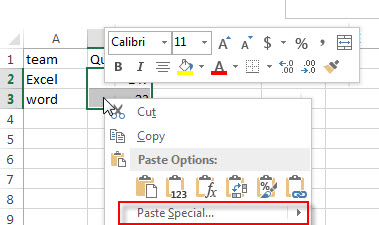4# the Paste Special window will appear.

5# select Multiply radio button from the Operation section.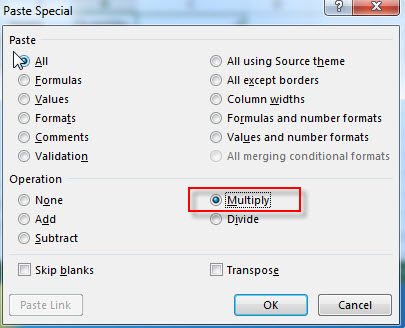6# Click OK button, you will see that all of the positive numbers should be change to negative.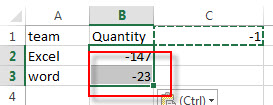If the selected range of cells contains negative numbers, then its values also will be multiplied by -1, so its signs also will be reversed as negative.

You can also write an Excel formula to multiply one negative number -1 to achieve the same result. the generic formula is like this:

`=B1* -1`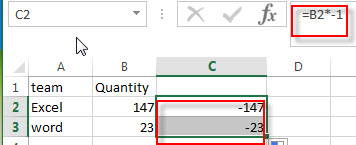## Convert Positive Numbers to Negative with VBA Code

You can also write a new excel macro to convert positive numbers to negative numbers in Excel VBA, just do the following:

1# click on “Visual Basic” command under DEVELOPER Tab.2# then the “Visual Basic Editor” window will appear.

3# click “Insert” ->”Module” to create a new module4# paste the below VBA code into the code window. Then clicking “Save” button.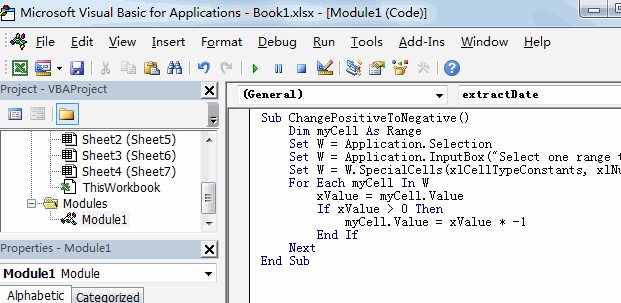```Sub ChangePositiveToNegative()
Dim myCell As Range
Set W = Application.Selection
Set W = Application.InputBox("Select one range that you want to change positive to negative:", "ChangePositiveToNegative", W.Address, Type:=8)
Set W = W.SpecialCells(xlCellTypeConstants, xlNumbers)
For Each myCell In W
xValue = myCell.Value
If xValue > 0 Then
myCell.Value = xValue * -1
End If
Next
End Sub```

5# back to the current worksheet, then run the above excel macro.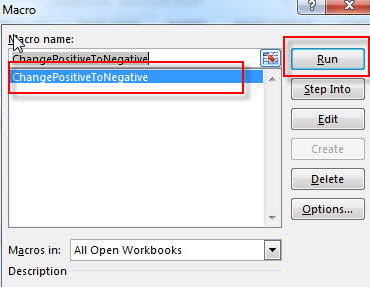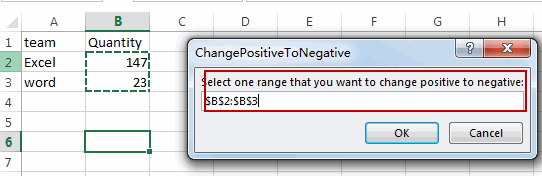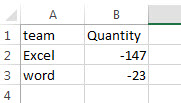Related Posts

VBA Macro For VLOOKUP From Another Sheet

In the previous post, you should know that how to fix or remove the #N/A error when using VLOOKUP formula to lookup value from another sheet. And this post will show you how to use VBA code to vlookup data ...

How To Insert Comments in Protected Worksheet in Excel

This post will show you how to allow comments in a protected worksheet in Excel. You can easily to insert comments into cells in a normal worksheet in Excel, but if want to insert a comment in a worksheet that ...

How To Convert Text to Upper Cases(Using VBA) in Excel

This post will show you how to switch from lower case to upper case in Excel. and I am going to show you two different ways of converting text to upper cases using formula or VBA macro in Excel 2013,Excel ...

How To Hide Every Other Row in Excel (Using VBA)

This post will show you how to hide alternate rows or columns in Excel or how to hide every third, fourth, fifth row or column in Excel. If you want to hide every other row in your current worksheet, how ...

How to Disable the Save As Prompt in Excel

This post will show you how to use a VBA Macro to save an Excel file and overwrite any existing file without a prompt so that you are going to get the little window that says file already exists do ...

How to Count Cells that Contain even or odd numbers in Excel

This post will guide you how to count the number of cells that contain odd or even numbers within a range of cells using a formula in Excel 2013/2016.How do I count cells that contain odd numbers through the use ...

How to Count Cells that Contain negative Numbers in Excel

This post will guide you how to count the number of cells that contain negative numbers within a range of cells using a formula in Excel 2013/2016.You can count the number of negative numbers in your data using easy functions ...

How to Count Cells Are Not Blank or Empty in Excel

This post will guide you how to count cells that are not blank or empty in a given range cells using a formula in Excel 2013/2016.How do I count the number of cells that are not blank in a particular ...

How to Count Cells Not equal to X or Y in Excel

This post will guide you how to count the number of cells not equal to criteria X or Y in a given range cells using a formula in Excel 2013/2016.You can easily to count cells equal to or not equal ...

How to Count Cells Less Than a Specific Value in Excel

This post will guide you how to count the number of cells less than a particular numeric value in a given range cells using a formula in Excel 2013/2016. How do I count cells that are less than a specific ...

Sidebar